# Questions: 1. Write a balanced equation for the reaction of zinc with hydrochloric acid, including states....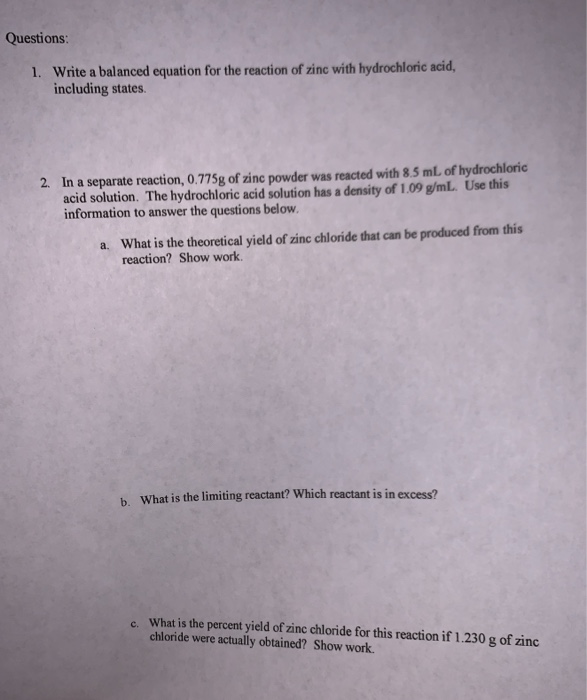Questions: 1. Write a balanced equation for the reaction of zinc with hydrochloric acid, including states. 2. In a separate reaction, 0.775g of zinc powder was reacted with 8.5 mL of hydrochloric acid solution. The hydrochloric acid solution has a density of 1.09 g/mL. Use this information to answer the questions below. a. What is the theoretical yield of zinc chloride that can be produced from this reaction? Show work. b. What is the limiting reactant? Which reactant is in excess? What is the percent yield of zinc chloride for this reaction if 1.230 g of zinc chloride were actually obtained? Show work.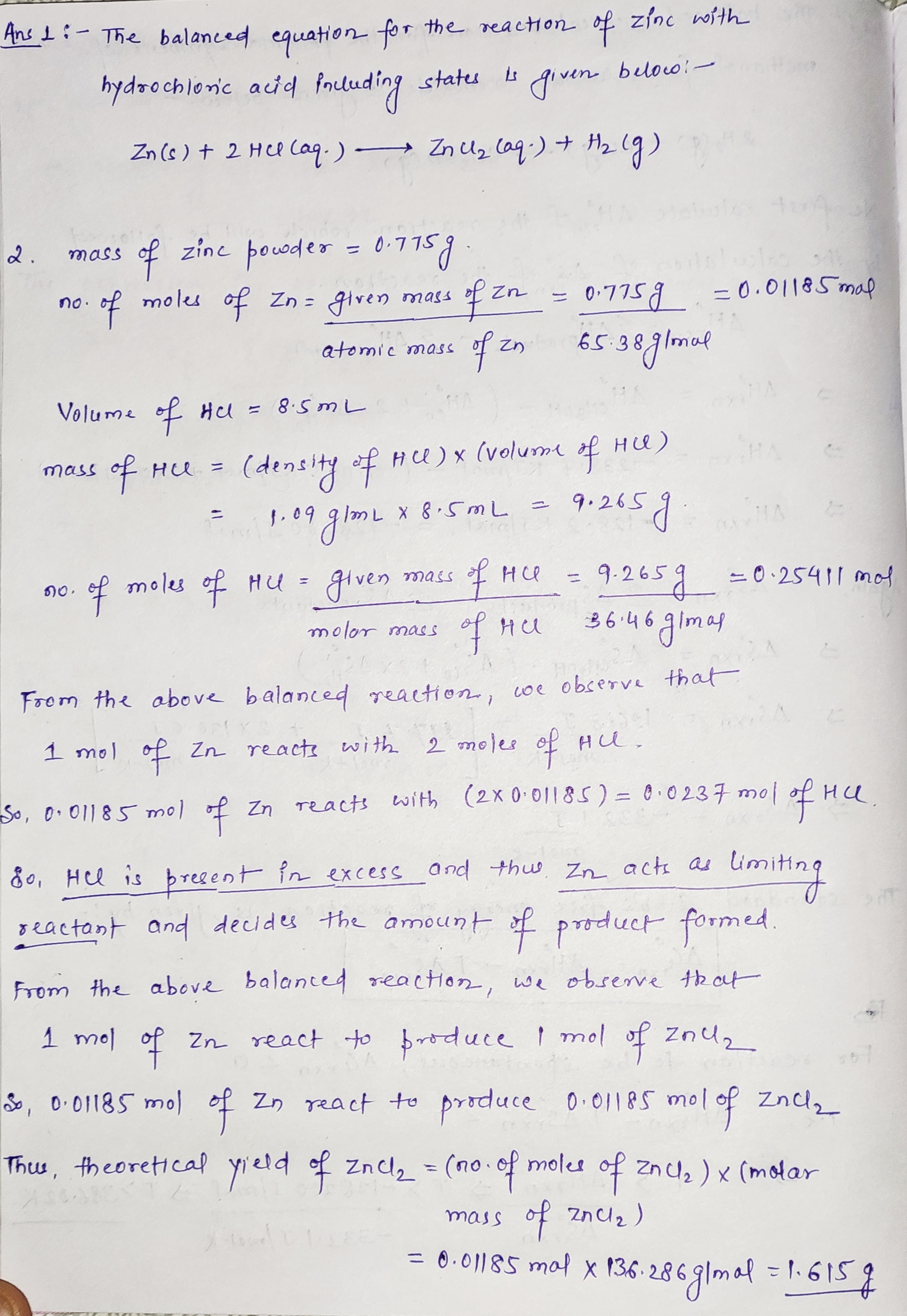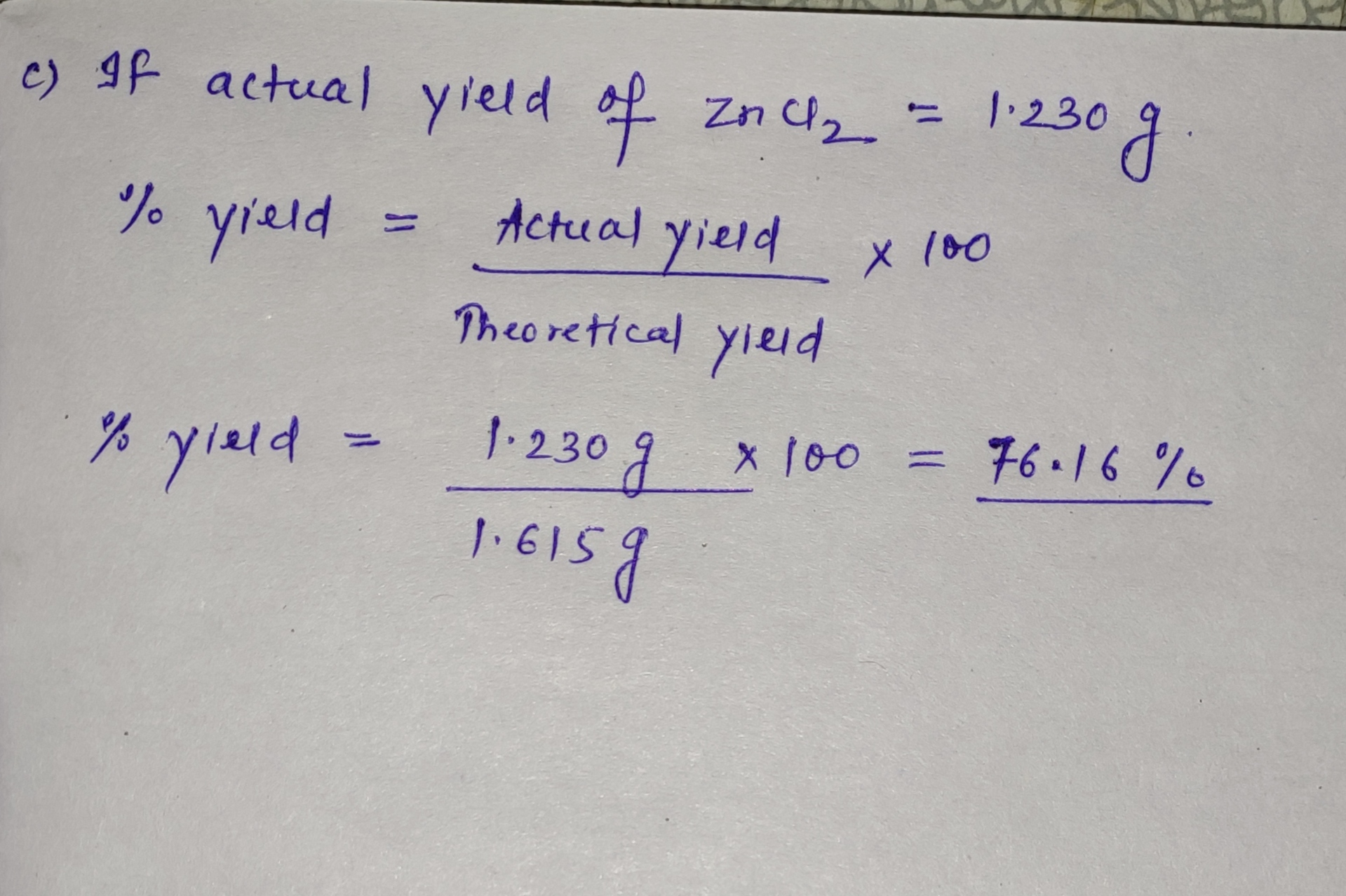#### Earn Coin

Coins can be redeemed for fabulous gifts.

Similar Homework Help Questions
• ### 1. What is meant by the term "limiting reactant"? 2. If some magnesium f some magnesium...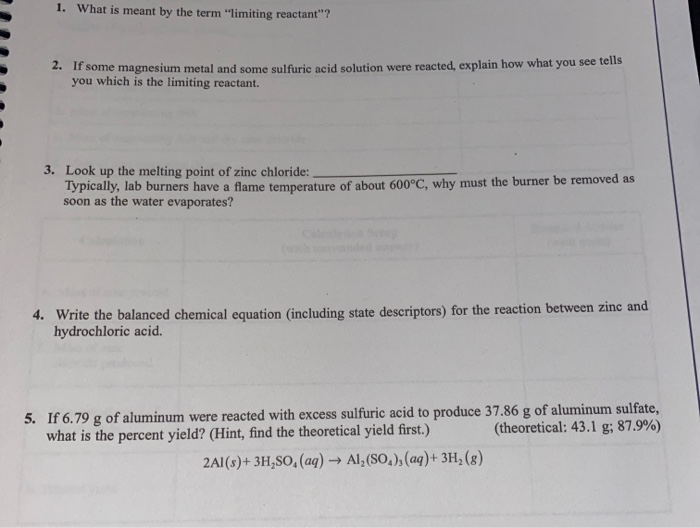1. What is meant by the term "limiting reactant"? 2. If some magnesium f some magnesium metal and some sulfurie neid solution were reacted, explain how what you see tells you which is the limiting reactant. 3. Look up the melting point of zinc chloride: Typically, lab burners have a flame temperature of about 600°C, why must the burner be removed as soon as the water evaporates? 4. Write the balanced chemical equation (including state descriptors) for the reaction between...

• ### Rion 1 of 3 > Chlorine gas can be prepared in the laboratory by the reaction of hydrochloric acid with manganese...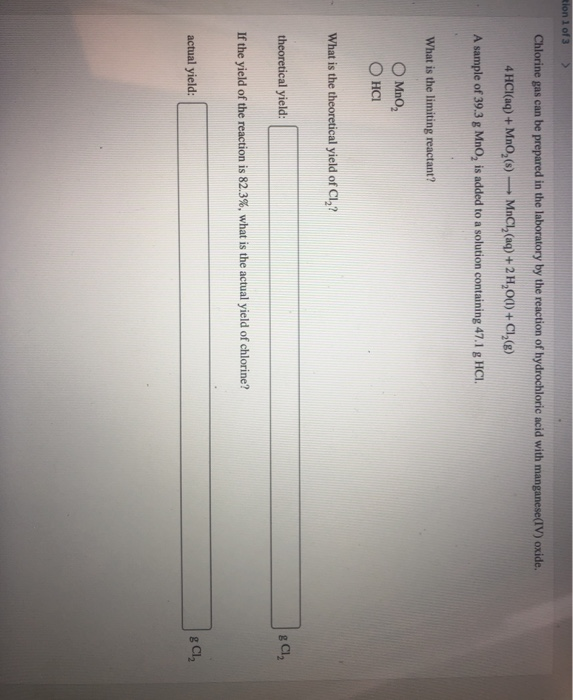Rion 1 of 3 > Chlorine gas can be prepared in the laboratory by the reaction of hydrochloric acid with manganese(IV) oxide. 4 HCl(aq) + Mn0,() — MnCl, (aq) + 2 H,0(1) + Cl,() A sample of 39.3 g Mno, is added to a solution containing 47.1 g HCI. What is the limiting reactant? Mno OHCI What is the theoretical yield of CI? theoretical yield: g Cl, If the yield of the reaction is 82.3%, what is the actual yield...

• ### A. Hydrochloric Acid Solution and pH 1. Write the balanced chemical reaction for the dissociation of...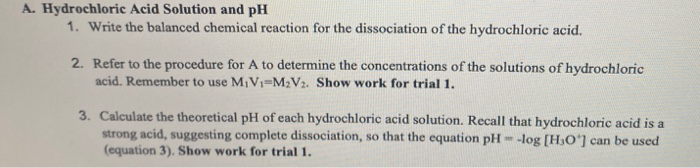A. Hydrochloric Acid Solution and pH 1. Write the balanced chemical reaction for the dissociation of the hydrochloric acid. 2. Refer to the procedure for A to determine the concentrations of the solutions of hydrochloric acid. Remember to use MiV1-MV. Show work for trial 1. 3. Calculate the theoretical pH of each hydrochloric acid solution. Recall that hydrochloric acid is a strong acid, suggesting complete dissociation, so that the equation pH log (HO) can be used (equation 3). Show work...

• ### Chlorine gas can be prepared in the laboratory by the reaction of hydrochloric acid with manganese(IV)...

Chlorine gas can be prepared in the laboratory by the reaction of hydrochloric acid with manganese(IV) oxide. 4HCl(aq)+MnO2(s)⟶MnCl2(aq)+2H2O(l)+Cl2(g) A sample of 34.5 g MnO2 is added to a solution containing 43.1 g HCl. What is the limiting reactant? What is the theoretical yield of Cl2? theoretical yield: g Cl2 If the yield of the reaction is 73.9%, what is the actual yield of chlorine? actual yield: g Cl2

• ### Write a balanced chemical equation for the reaction between calcium chloride and potassium carbonate. Using stoichiometry,...

Write a balanced chemical equation for the reaction between calcium chloride and potassium carbonate. Using stoichiometry, calculate the limiting reactant and the theoretical yield (the mass of CaCO3 product) for the reaction. What is the actual yield of CaCO3(s) formed from your experiment? Show your work. Determine the percent yield of the reaction.

• ### Chlorine gas can be prepared in the laboratory by the reaction of hydrochloric acid with manganese(IV)...

Chlorine gas can be prepared in the laboratory by the reaction of hydrochloric acid with manganese(IV) oxide. 4HCl(aq)+MnO2(s)⟶MnCl2(aq)+2H2O(l)+Cl2(g) A sample of 36.7 g MnO2 is added to a solution containing 48.9 g HCl. What is the limiting reactant? MnO2 HCl What is the theoretical yield of Cl2? theoretical yield: g Cl2 If the yield of the reaction is 72.1%, what is the actual yield of chlorine? actual yield:

• ### 4. Zinc metal reacts with hydrochloric acid according to the balanced equation Zn(s) + 2 Ha...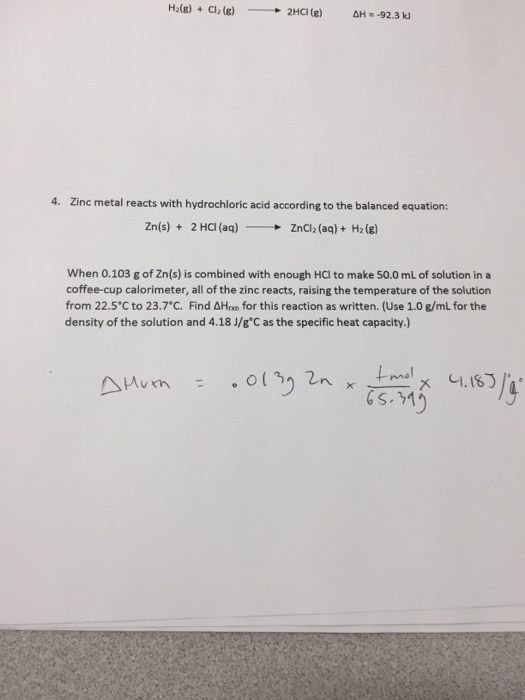4. Zinc metal reacts with hydrochloric acid according to the balanced equation Zn(s) + 2 Ha (aq) ZnCl2 (aq) + H2 (g) - → When 0.103 g of Zn(s) is combined with enough HCI to make 50.0 mL of solution in a coffee-cup calorimeter, all of the zinc reacts, raising the temperature of the solution from 22.5°C to 23.7°C. Find 솨lan for this reaction as written. (Use 1.0 g/mL for the density of the solution and 4.18 J/g°C as the...

• ### 10. Write the balanced equation for the neutralization reaction between a) hydrochloric acid and potassium hydroxide...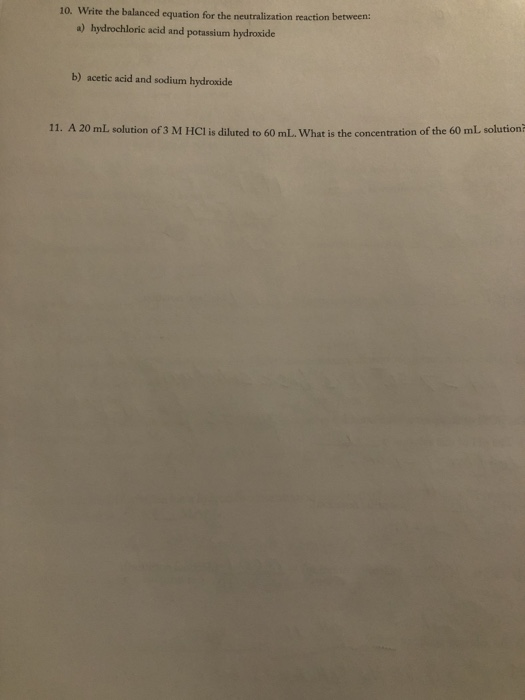10. Write the balanced equation for the neutralization reaction between a) hydrochloric acid and potassium hydroxide b) acetic acid and sodium hydroxide 11. A 20 mL solution of 3 M HCl is diluted to 60 mL. What is the concentration of the 60 ml solution LAPUUNLI Chemical Properties of Compounds: Chemical Reactions Questions and Problems (May be assigned before or after the experiment, depending on your instructor.) (Show all calculations where appropriate.) 1. A room temperature white solid is mixed...

• ### Chlorine gas can be prepared in the laboratory by the reaction of hydrochloric acid with manganese(IV)...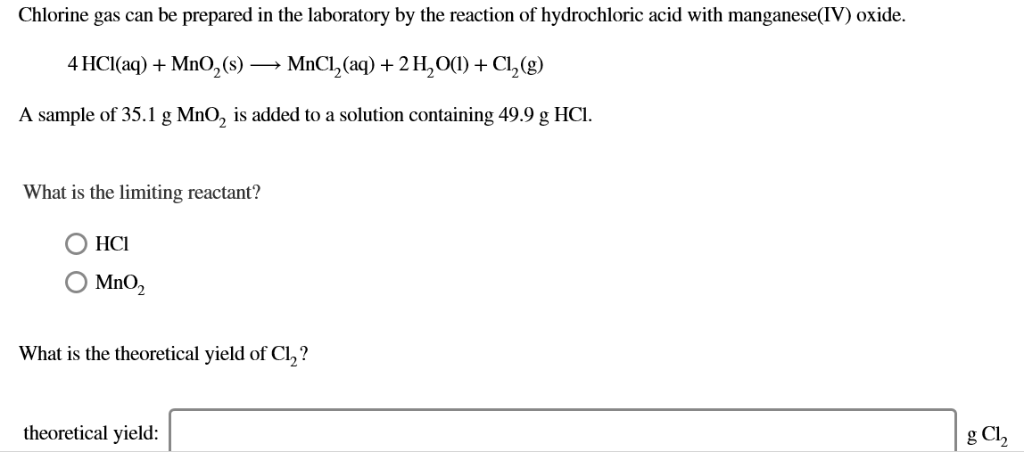Chlorine gas can be prepared in the laboratory by the reaction of hydrochloric acid with manganese(IV) oxide 4 HCl (aq)MnO2(s) MnCl2 (aq) 2H2O(C) + Cl2(g) A sample of 35.1 g MnO, is added to a solution containing 49.9 g HCI. What is the limiting reactant? O MnO, What is the theoretical yield of Cl,? theoretical yield: g Cl, What is the theoretical yield of Cl,? theoretical yield: g Cl2 If the yield of the reaction is 71.7%, what is the...

• ### Chlorine gas can be prepared in the laboratory by the reaction of hydrochloric acid with manganese(IV)...

Chlorine gas can be prepared in the laboratory by the reaction of hydrochloric acid with manganese(IV) oxide. 4HCl(aq)+MnO2(s)⟶MnCl2(aq)+2H2O(l)+Cl2(g) A sample of 43.7 g MnO2 is added to a solution containing 49.3 g HCl. What is the limiting reactant? HCl MnO2 What is the theoretical yield of Cl2? theoretical yield: g Cl2 If the yield of the reaction is 87.3%, what is the actual yield of chlorine? actual yield: g Cl2